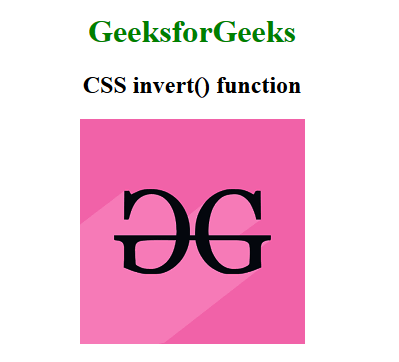# CSS | invert() Function

The invert() function is an inbuilt function that is used to apply a filter to the image to set the invert of the color of the sample image.

Syntax:

`invert( amount )`

Parameters: This function accepts single parameter amount which holds the amount of conversion. The value of invert is set in terms of value and percentage. The value 0% represents original image and 100% represents the inverted image.

The invert() function internally uses the following formula, to computer the inverse of the image:

`amount * (255 - value) + (1 - amount) * value`

The value of inversion is controlled by the variable `amount`, the variable value lies between 0 – 1(floating) range (this is done by converting the passed percentage of color inversion to a value between 0-1). The value is the the color value of the pixel. `(255-value)` gives the color after subtracting the color value with the max pixel value, assumes that the value of the pixel is in the range 0 – 255 (though input image sample space could be stretched/scaled to meet the specified criteria).

Below is a table containing a list of inversion percentages and the result they produce.

Inversion Result
0% Original Image
50% Image with each pixel having grey color
100% Completely inverted Image

Below example illustrates CSS invert() function in CSS:
Example:

 `  ` `<``html``>  ` ` `  `<``head``>  ` `    ``<``title``>CSS invert() Function  ` `     `  `    ``<``style``> ` `        ``h1 { ` `            ``color:green; ` `        ``} ` `        ``body { ` `            ``text-align:center; ` `        ``} ` `        ``.invert_effect { ` `            ``filter: invert(100%); ` `        ``} ` `    `` ` `  ` ` `  `<``body``>  ` `    ``<``h1``>GeeksforGeeks  ` `     `  `    ``<``h2``>CSS invert() function ` `     `  `    ``<``img` `class``=``"invert_effect"` `src``=  ` `"https://media.geeksforgeeks.org/wp-content/cdn-uploads/20190710102234/download3.png"` `        ``alt``=``"GeeksforGeeks logo"``>  ` `  ` ` `  ` `

Output:Supported Browsers: The browsers supported by invert() function are listed below:

• Internet Explorer
• Firefox
• Safari
• Opera

My Personal Notes arrow_drop_upCheck out this Author's contributed articles.

If you like GeeksforGeeks and would like to contribute, you can also write an article using contribute.geeksforgeeks.org or mail your article to contribute@geeksforgeeks.org. See your article appearing on the GeeksforGeeks main page and help other Geeks.

Please Improve this article if you find anything incorrect by clicking on the "Improve Article" button below.

Improved By : deosurya2019

Article Tags :

Be the First to upvote.

Please write to us at contribute@geeksforgeeks.org to report any issue with the above content.